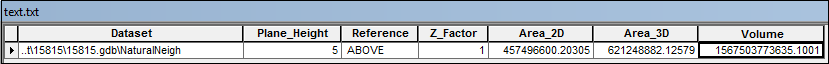English

# How To: Calculate the volume of a region from elevation points

## Summary

Elevation points represent the height of the land surface and each point contains the x,y,z measurements of the earth surface. The volume of a region can be calculated with elevation points as the input, and the results give information such as how much soil is collected from excavating a certain area of land to a specific depth. The volume of a region can be calculated from the elevation points by interpolating a raster surface from the elevation points, and running the Surface Volume tool to calculate the volume of the region above or below a specific elevation (reference plane).

```Note:

The instructions provided describe how to calculate the volume of a region from elevation points.

## Procedure

1. In ArcMap, add the elevation points and boundary feature classes.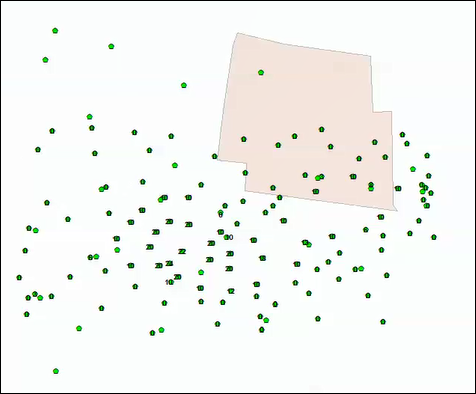2. Interpolate a raster surface from the elevation points using the Natural Neighbor tool.
```Note:
There are several interpolation methods available in ArcGIS Desktop depending on the purpose of the raster interpolation. For more information on other raster interpolation methods, refer to An introduction to interpolation methods.```
1. Navigate to 3D Analyst Tools > Raster Interpolation > Natural Neighbor.
2. Select the elevation points feature class as the Input point features.
3. Select the field holding the height value for each point as the Z value field.
4. Set a name and location for the Output raster.
5. Click OK. The interpolated raster is displayed in the map.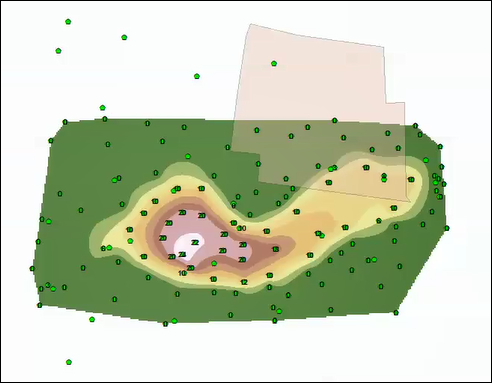3. Cut out the raster created in Step 2 with the boundary as the output extent using the Clip tool.
```Note:
In this scenario, the boundary feature class represents the area of interest, not the entirety of the interpolated area.```
1. Navigate to Data Management Tools > Raster > Raster Processing > Clip.
2. Select the raster created in Step 2 as the Input Raster.
3. Select the boundary layer as the Output Extent.
4. Check the check box for Use Input Features for Clipping Geometry.
5. Set a name and location for the Output Raster Dataset.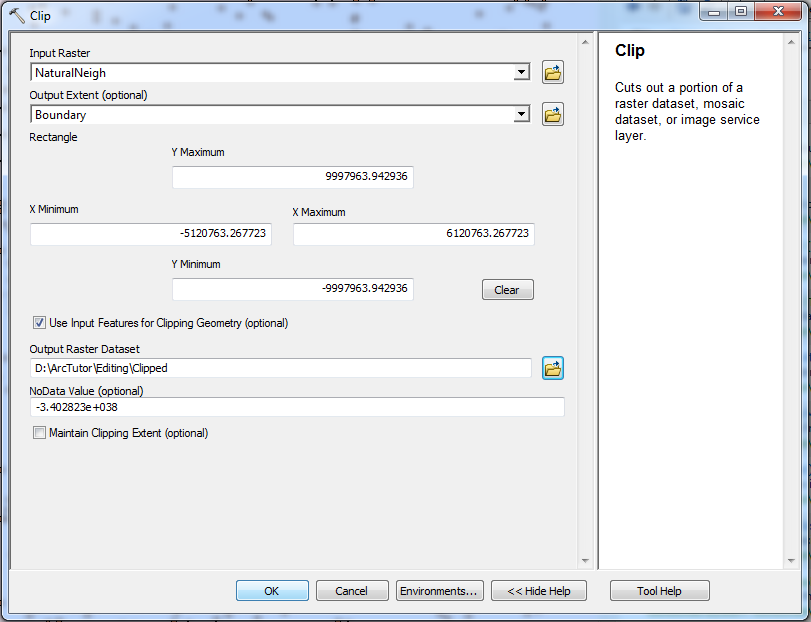6. Click OK. The clipped raster is displayed in the map.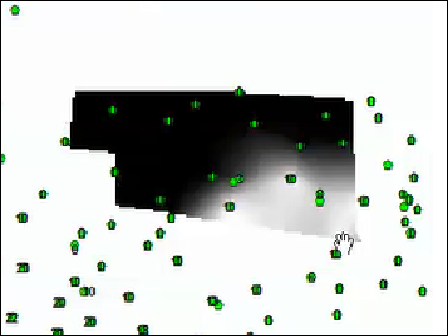4. Calculate the surface volume of the region using the Surface Volume tool.
1. Navigate to 3D Analyst Tools > Functional Surface > Surface Volume.
2. Select the clipped raster in Step 3 as the Input Surface.
3. Set a name for the Output Text File.
4. Select ABOVE or BELOW for the Reference Plane to calculate the volume between the specified plane height and the portions of the surface that are above or below the reference plane.
5. Insert the Z-value of the plane to be used as the base to calculate the volume in the Plane Height parameter.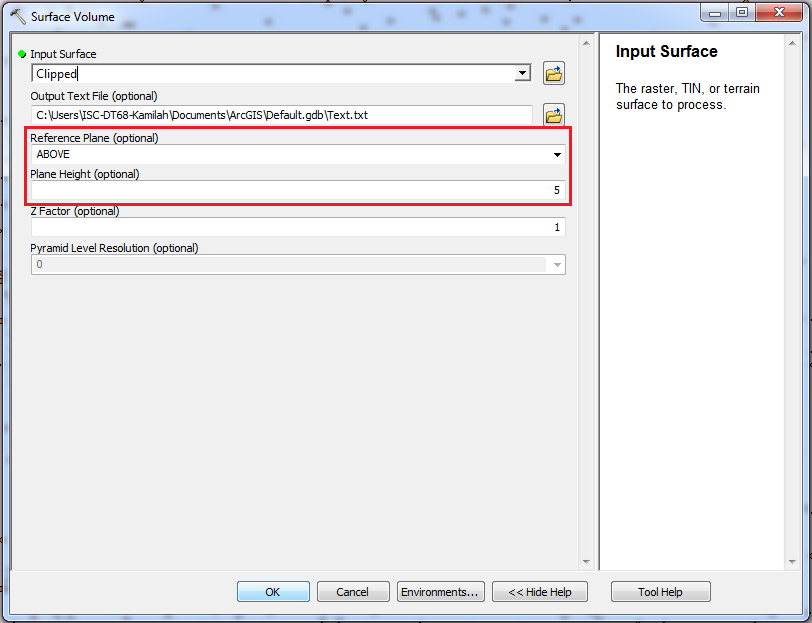6. Click OK. The output text file stores the full path to the surface, the parameters used to generate the results, and the calculated area and volume measurements.